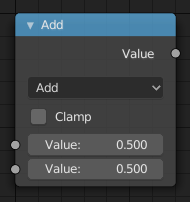# Math Node¶Math node.

The Math Node performs math operations.

## Inputs¶

Value
First numerical value. The trigonometric functions accept values in radians.
Value
Second numerical value. This value is not used in functions that accept only one parameter like the trigonometric functions, Round and Absolute.

## Properties¶

Operation
Add, Subtract, Multiply, Divide, Sine, Cosine, Tangent, Arcsine, Arccosine, Arctangent, Arctangent2, Power, Logarithm, Minimum, Maximum, Round, Less Than, Greater Than, Modulo, Absolute
Clamp
Limits the output to the range (0 to 1). See clamp.

## Outputs¶

Value
Numerical value output.